# How to use the Clean and Define tool

With the clean and define tool you have access to a number of features that a data processing specialist normally will need to do in a workflow.

The User interface is optimized to allow the data specialist to work with an XML editor to manipulate the data structures directly. The beauty of XML is that it is simple to edit and copy. The disadvantages are that you need some knowledge of XML and you must be careful to avoid creating errors.

Validation of XML to ensure that it is properly structured can be a substantial effort for a computer to perform but it is always a good idea to take the time to sanity check the data after a clean run in order to see that the process has been performed correctly. A little effort at this stage will pay off handsomely in time saved later.

The three different levels of a clean document
The clean and define tool works at three different levels. You may choose to perform them in any order, but the order should always be the same. The processes are performed sequentially:

1. Structure
• Select-statements
• Create statements
2. Groups
• Set commands
• Arithmetic commands
3. Weighting

As soon as you have created a clean tool, an XML template will open. You can modify this in order to create a cleaning tool. Note: Remember to specify an input data set.Structure

The structure commands will select which questions you want to bring into the cleaning process and consequently into the final data set. The select statements require knowledge of the Research Studio address syntax.

Select-statements

Here are some typical select statements:

• <select a=’\Q1,,Q5’ /> (select all questions from Q1 to Q5)
• <select a=’\Q1,Q3,Q5’ /> (select questions Q1,Q3 and Q5)
• <select a=’\Q1.a,Q5.b’ /> (select first sub-question in Q1 and second sub-question in Q5)
• <select a=’\Q1.a.1,,6,8’ /> (select rows from 1 to 6 and 8 from question Q1)
• <select a=’\Q1,,QNN’ /> (select all questions from Q1 to QNN, where QNN is the last question in the data set. This will bring all questions into the data set.)

Note: If you want all questions to be included in the new data set the select statements are unnecessary.

You may also write multiple select statements if you wish to select parts of different questions as shown in the example below.

An example:

The following XML will create new questions with only sub-sets of question S1 in our example:



Special select statements

The select element has three attributes that can also modify the question. They are “transpose”, “convert”, and “concat”.

• <select a=’\5.b’ transpose=”true” />

Will transpose a selected Grid-question to one single or multiple type question for each row of the grid.

 From this To this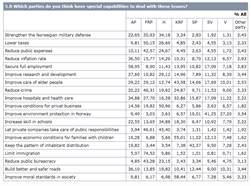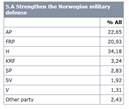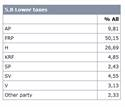• <select a=’\7.b’ convert=”true” />ll convert a selected Grid-question to one question for each COLUMN
 From this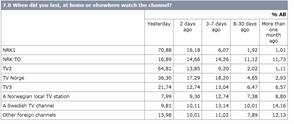To this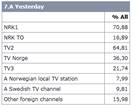• <select a=’\3,4’ concat=”r” />

Will concatenate all questions selected into one single question with multiple rows.

• Specifying concat=”s” will concatenate into multiple sub-questions
 From this To this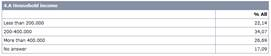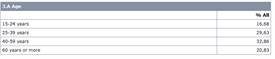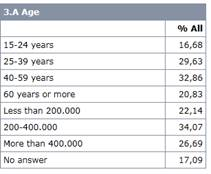Note that the label from the first question will be used for the concatenated question. This can be overridden when performing an analysis using Research Analyzer, or the text may be edited in the XML file directly or a questionnaire match (as described earlier) can be used.

A final select statement that can be useful is when you want to merge rows, e.g. in a brand tracker where there previously were three different sub-brands tracked and now only the master brand was tracked.

• <select a=’\5.b.1:3,4’ />

Will merge rows 1 to 3 into one category. This may imply that a single grid question has more than one answer

 From this To this• <select a=’\7.b’ convert=”true” />

Will convert a selected Grid-question to one question for each COLUMN

 From this To this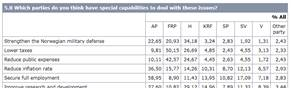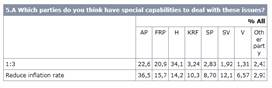Note that the labels will be replaced with the codes. This can be overridden when performing an analysis using Research Analyzer, or the text may be edited in the XML file directly or a questionnaire match (as described earlier) can be used.

Other examples

Using address syntax to select and rearrange

Example 1

Select one or more sub-questions or rearrange the order of sub-questions within a question: <select a=”\Q1.B,E,A”/> selects Q1 sub-question B, then E, then A.

Example 2

Select one or more rows and re-arrange the order of the rows:
<select a=”\Q1.3,1,2,4,,8”/> selects Q1 row 3, then 1, then 2 then rows 4, 5, 6, 7, 8

Example 3

Select one or more columns of a grid single/multi question and re-arrange the order:
<select a=”\Q1.A.1,,5[3,2,1,4,,6]”/> selects Q1, sub-question A, rows 1,2,3,4,5 column 3, then 2, then 1, the 4, 5, 6.

Inserting new rows

Example 4

Insert new rows or columns in the data structure of a question: <select a=”\Q1.A.1,,3,!,4,,8[1,,3,!,4,5]”/> selects Q1, sub-question A, rows 1, 2, 3, then insert an empty row, then row 4, 5, 6, 7, 8, and assuming this is a grid single/multi pick columns 1, 2, 3, then insert an empty column, and finally columns 4 and 5.

Using the convert attribute to change between grid questions and multiple sub-questions.

Example 5

Select a grid single/multi question and convert it to multiple multi-choice sub-questions:
<select a=”\Q1.A” convert=”yes”/>

Select a single or multi-choice question and convert it into a single multigrid question
<select a=”\Q1.A,B,C” convert=”yes”/>

These two examples look nearly the same. The main difference between them is the type of question that they start with.

Transposing rows and columns

Example 6

Transpose the rows and columns of a single grid or multigrid question. The resulting question will be a multigrid.
<select a=”\Q1.A” transpose=”yes”/>

Perform a logical OR combination on question elements

Example 7

In another variation of address syntax, you may combine either rows or columns using a logical OR operation.

“OR” rows into one:

<select a=”\Q1.A.1,3,2,4:5,8,7;9;10”/> would select Q1, sub-question A, row 1 then 3, then 2, then “OR” rows 4 and 5 into 1 new row, then 8, then “OR” rows 7, 9 and 10 into one row.

Any text for combined rows, will be a string that is just the “OR” operation performed. From the example above, text for the two newly created rows should be “4:5” and “7;9;10”. If you desire different texts you may create a new questionnaire from the output dataset and edit it there.

When combining rows you should use caution. For single response type questions, you can cause multiple answers. Single grid questions types will likely have multiple answers for “OR”ed rows. Numeric or time type questions will have the values from each row added. Be sure to test how the “OR” operation effects your data to ensure consistency.

“OR” columns:

Columns in single or multiple grids can be combined using the “OR” operation.
<select a=”\Q1.A.1,,5[2,1,3:4,7;10]”/> will select Q1, sub-question A, rows 1, 2, 3, 4, 5 columns 2, then 1, then “OR” columns 3 and 4 and finally “OR” columns 7 and 10.

Any text for combined columns will be a string with a copy of the “OR” operation performed. From the example above, text for the two newly created columns should be “3:4” and “7;10”. To change the text, use the method suggested when “OR”ing rows above.

“OR” single/multi sub-questions into one:

<select a=”\Q1.B:C”/> will select Q1, and “OR” sub-questions B and C into a single sub-question. The new sub question text will be from the first sub-question selected and the type will be set to multiple answer. All rows will be joined as it is not possible to select a subset of rows.

Concatenate rows from several questions

Example 8

The concatenation attribute is used to combine across sub-questions. <select a=’\Q1,Q2,Q4’ concat=”r”/> will create a new question called Q1, with all rows from questions Q2 and Q4 appended.

All questions must have the same structure (same number of sub-questions, type and number of columns). It is possible to cause multiple answers in single answer questions using this operation. Be sure to check the output data for inconsistencies.

Concatenate sub-questions from several questions into one:

Example 9

<select a=’\Q1,Q3,Q5’ concat=”s”/> will create a new question called Q1 with all sub-questions from Q3 and Q4 added to the first.

All questions must have the same number of rows. Sub-question text and underlying structures are taken from each sub-question. Text for each row is taken from the first question.

Create statements

Previously we have described a method for creating “empty questions” using the questionnaire matcher. Normally this will be the simplest way of defining new questions.

You may also create new questions directly in the Clean and define tool, and this requires that you write this in the xml format shown in the template.

To perform these tasks you should have knowledge of the xml-coding of questionnaires. The code may also be copied from a question made in the questionnaire editor.

However, for creation of new and derived variables, we recommend that you use the questionnaire editor with access to all features from libraries etc.

The template for creating a new single question in the clean tool (it might be the quickest way if there are only a minor added question).

<create>
<ques id="new">
<subq type="n">
<stext>
<text>Question text</text>
</stext>
</subq>
<rgroup>
<r>
<text>Row 1</text>
</r>
<r>
<text>Row 2</text>
</r>
</rgroup>
</ques>
</create>

Cleaning – Set and Arithmetic commands

Cleaning is done using a series of <group> elements containing any number of <arithmetic> and <set> elements. The statements will be executed in sequence, so that later statements can use results of previous ones.

How to use <arithmetic>

The <arithmetic> tag is used to perform calculations based on certain conditions. A“%” sign plays the part of a placeholder for the row number. Statements containing “%” sign will be executed for all rows in a question.

Attributes for the <arithmetic> tag:

 Name Description condition Defines a filter that will trigger an arithmetic operation. action Defines a filter that performs the arithmetic operation. location Location where you wish to place the results of the arithmetic operation. useCatCodeValues If set to ”true”, the values of the locations defined in the condition and/or action attributes will be used for the arithmetic operation.

Examples using the arithmetic

Note that when using the <arithmetic> element, care must be taken to ensure that operations make sense and do not attempt to mix question types unreasonably.

Example 1

Check the value in each row of Q17 sub-question b to see if it is equal to 0 through 5. If so, multiply that value by the value found at the corresponding row of Q18 sub-question a and place the result in the corresponding row of question Q19 sub-question a.

<arithmetic condition="\Q17.b.%=0:5" action="\Q17.b.%*\Q18.a.%" location="\Q19.a.%" useCatCodeValues="true" />

The values found at the row locations are used because “useCatCodeValues” is set to true.

Example 2

Check the selection in each row of Q17 sub-question b to see if it is in column 0 through 5. If so, set the corresponding row of Q19 sub-question a equal to the setting of the question Age sub-question a row 1.

<arithmetic condition="\Q17.b.%=0:5" action="\Age.a.1" location="\Q19.a.%" />

You may also insert a serial number into any numeric question.

<arithmetic action="serial" location="\Q.S.R" condition="..." />

where \Q.S.R is a reference to an existing or tool created numeric question (F), condition is an optional logical filter.

This statement will fill \Q.S.R with serial numbers starting from 1.

Using <set>

The <set> tag is used in an IF/THEN manner. If the condition is matched, perform the action defined.

Attributes for the <set> tag:

 Name Description condition Defines a filter that will trigger an arithmetic operation. action Defines a filter that performs the arithmetic operation.

Example 1

For every row of Q1 sub-question a, check to see if it is selected. If it is, Q2 will be set to the corresponding option.

<set condition="\Q1.a.%=1" action="\Q2=%" />

Weighting
One or more weigh set definitions can be created. The same syntax as used in Research Analyzer weighting documents should be used. Note: The weighting can be done more easily in Research Studio using the “Weight Creation Wizard”.

<weights> - Starts a weight document
Root element of the weight document and has no attributes. Can have one or more weight sets.

< wset > - Starts a weight set
An introduction to a weight set. A <wset> element consists of at least one or more <rim> elements.

decimals

The total population will be shortened according to this.

 N Specify the number of decimals the total population should be shortened with.

Example: In the following syntax <wset pop='3500123' decimals='3'> the total will be shown in the tables as 3500.

Id

Specify an ID for this weight set.

 name Specify the weight set name. The name should be unique within this dataset.

Example: A specified weight set is called 'wsetA' with the syntax <wset id='wsetA'>. In the analysis document, this weight set may be called as follows: <xt a='\Q1' wset='wsetA'/>.

pop

Describes the total population for the weight set.

 N Specify the total value which the weights should add up to. R The total population adds up to the sum of all respondents (items). ? The total population will add up to the sum of all pop='nnn' given in the different 's in the element. If a weight set consists of MORE than one element, the value '?' will take the sum from the FIRST rim.

preweights

Specify a preweight

 addr Specify a QUANTITATIVE variable which should be used as a preweight for this weightset.

Note: If you only want to make a weight based on a quantitative variable in the dataset, you can specify a <rimcells> with all respondents, and address the quantitative address as a preweight.

< text > - Text that specifies the population row
Create the text that shall occur in the Population row (poprow) in the tables. This element has no attributes. Example: <text>Tot. number of population:</text> in the weights document will create the text 'Tot. number of population:' in the population row in each WEIGHTED table created in the analysis. This can later be overrided with poprow='no' in the analysis syntax

< rim > - Starts a weight rim.
An introduction to a weight rim. A <rim> element consists of at least one or more <rimcells>.

Type

The total population will be shortened according to this.

 factor The total population in the 's will be the sum of all respondents in the respective multiplied with their relative preweights. target The total population will add up to the total specified in the population attribute (pop='xxxx') in the rimcell.

Example (one rim weighting):

<weights>
<wset id="GenRegAge" pop="3547" decimals="0">
<text>Population</text>
<rim>
<rimcell expr="\1.A=1&\2.A=1&\3.A=1" pop="148"/>
<rimcell expr="\1.A=1&\2.A=1&\3.A=2" pop="282"/>
<rimcell expr="\1.A=1&\2.A=2&\3.A=1" pop="73"/>
<rimcell expr="\1.A=1&\2.A=2&\3.A=2" pop="120"/>
<rimcell expr="\1.A=1&\2.A=3&\3.A=1" pop="38"/>
<rimcell expr="\1.A=1&\2.A=3&\3.A=2" pop="65"/>
<rimcell expr="\1.A=1&\2.A=4&\3.A=1" pop="26"/>
<rimcell expr="\1.A=1&\2.A=4&\3.A=2" pop="45"/>
<rimcell expr="\1.A=2&\2.A=1&\3.A=1" pop="144"/>
<rimcell expr="\1.A=2&\2.A=1&\3.A=2" pop="275"/>
<rimcell expr="\1.A=2&\2.A=2&\3.A=1" pop="69"/>
<rimcell expr="\1.A=2&\2.A=2&\3.A=2" pop="113"/>
<rimcell expr="\1.A=2&\2.A=3&\3.A=1" pop="37"/>
<rimcell expr="\1.A=2&\2.A=3&\3.A=2" pop="60"/>
<rimcell expr="\1.A=2&\2.A=4&\3.A=1" pop="24"/>
<rimcell expr="\1.A=2&\2.A=4&\3.A=2" pop="42"/>
</rim>
</wset>
</weights>

Example (two rims weighting):

<weights>
<wset id="TwoRims" pop="R" decimals="0">
<text>Age/Gender</text>
<rim>
<rimcell expr="\1.A=1" pop="49"/>
<rimcell expr="\1.A=2" pop="51"/>
</rim>
<rim>
<rimcell expr="\2.A=1" pop="73"/>
<rimcell expr="\2.A=2" pop="120"/>
<rimcell expr="\2.A=3" pop="38"/>
<rimcell expr="\2.A=4" pop="65"/>
</rim>
</wset>
</weights>

Weighting general description:

Sometimes in surveys, we treat the respondents as representatives of the total population of which they are a sample. Normally, tables reflect the attitudes of the people interviewed, but we may want the tables to reflect the attitudes of the total population instead so that it seems as if we had interviewed everyone rather than just a sample of the population. This, of course, assumes that the people interviewed are a truly representative sample.

If we take a sample of 380 from a population of 10,000 middle-aged housewives and discover that 57 members of this sample buy cheddar cheese, we may want the number of middle-aged housewives who buy cheddar cheese to read 1,500 in our tables, not 57.

Moving from 57 to 1,500 is the fine art of weighting. In this case, each middle-aged housewife

has a weight of 10,000/380. Since 57 of them buy cheddar cheese, the number in the cell will be:  10000 / 380 ´ 57 = 1,500

Weighting is also used to correct biases that build up during a survey. For example, when conducting interviews by telephone you may find that 60% of the respondents were women. You may then want to correct this ratio of men to women to make the two groups more evenly balanced.

The basic idea behind weighting is that when someone falls into a given cell (that is, satisfies the conditions for that cell) the number in the cell is not increased by 1; rather, it is increased by 1 multiplied by the individual’s weight.

Weighting methods

Analyzer is sufficiently flexible to allow more than one set of weights for a given set of respondents. Which set is applied is determined by options.

Each set of weights, however, will apply one weight for each respondent. There are two ways of calculating weights:

•   The weight for each respondent may be part of the data for that respondent, or it may be calculated in the edit and passed to the tabulation section as a variable. You typically activate such weights on import.
•   The more common method of weighting is to define a set of characteristics and apply specific weights to respondents satisfying those characteristics.
• Our example above uses characteristic weighting, where the characteristics are age, sex, and working status. Thus, all respondents who are women aged between 45 and 54 and who don't work outside the home receive a weight of10,000/380.

The characteristics must be such that each record satisfies one unique set. Each respondent falls into one, and only one, set and no respondent is left out. Because of this, you must check all columns containing the characteristics and if necessary, correct any errors. For example, if one characteristic is sex and it is coded in column 6 of card 1, with a code of 1 for male and 2 for female, you must make sure that c106 is single coded with a ‘1’ or a ‘2’ only. It must not be blank, multi coded or otherwise miscoded in any way.

Analyzer offers factor, target, and rim weighting, preweights, weighting using proportions and weighting to a given total. These are described, with examples, in the sections which follow. The keywords used to write the weighting matrices are described later in this chapter.

Factor weighting

With factor weighting, every record which satisfies a given set of conditions is assigned a specific weight. You would generally use it when the weights are calculated outside of Analyzer — for instance, you may be told that all unemployed people in London require a weight of 10.5, whereas unemployed people in the rest of the country need a weight of 7.3.

When Analyzer creates the weighted table, it will check which cell of the weighting matrix each respondent belongs in, and will apply the weight associated with that cell before placing the respondent in the table.

You can also use factor weighting, with a factor of 1.0, when you just want to use weights stored

in the data or calculated in the edit, without defining any other weights. These weights are defined as preweights.

Target weighting

Target weights may be used when you know the exact number of respondents you want to appear in each cell of the weighted table. For example, in a table of age by sex, you may know the exact number of men under 21, women under 21, and so on, to appear in the table once it has been weighted. The weights that you define in your matrix are therefore the values to appear in the weighted table rather than the weights to be applied to each respondent of a given age and sex.

When Analyzer creates your weighted table, it calculates the weight for an individual respondent by taking the target figure for the appropriate cell in the weight matrix and dividing it by the number of respondents in that cell.

As an example, suppose that you have three groups of people. The first contains 100 people, the second contains 200, and the third contains 300. You know that in the total population, the spread of any 600 respondents across these three groups would be 150, 200, and 250. When Analyzer finds someone in the first group it will apply a weight of 1.5 (150/100) in order to obtain the total of 150 respondents in the weighted table. Respondents in the second group will have a weight of 1.0 because the number of respondents in this group matches the value in the weighting matrix for that group. Respondents in the third group will have a weight of 0.83 (250/300) because there are more people in that group than in the corresponding cell of the weighting matrix.

In this example, the number of people in our three groups was the same as the population defined in the weighting matrix. This will not always be the case. Often you will find that the values in the weighting matrix add up to more or less than the number of people you have in your sample. For instance, the spread of the population across your three groups may be 150, 250, and 250, giving a total of 650 respondents. When Analyzer balances your sample, it will weight each respondent according to the values in the matrix so that the total number of respondents in your weighted table will be 650, rather than the 600 that were interviewed.

If you decide that you want the total in the weighted table to be the same as the total number of respondents in your sample, you may define this total as part of the weighting matrix using the keyword total= which is described below. When Analyzer reads this keyword it balances the three groups according to the weights in the matrix and then adjusts all three weights so that the weighted total is 600.

Another variation of target weighting occurs when instead of knowing the actual number of people in each group of the population, you know that each group is a given percentage of the population.

For instance, the first group may be 27% of the population, the second may be 48%, and the third maybe 25%. In cases like this, you include the keyword input (see below) in the weighting matrix with the percentages for each group.

Rim weighting

Rim weighting is used when:

•   You want to weight according to various characteristics, but do not know the relationship of the intersection of those characteristics,or
•   You do not have enough respondents to fill all the possible cells of the table if you were to weight the data using the multidimensional technique described above.
• For example, you may want to weight by age, sex and marital status and may know the weights for each category of those characteristics; for example, people aged 25 to 30, men, single people.

However, you may not know the weights for, say, single men aged between 25 and 30, married women aged between 31 and 40, and so on.

On another study, you may need to weight by a large number of characteristics at the same time; for example, sex, age, race, occupation and income. Since each of these characteristics will be broken down into categories, you will require a weighting matrix with many cells. You may not have enough information to write a standard multidimensional weighting matrix which defines weights for the intersection of all these characteristics. However, as long as you have information on each category individually (for example, male, female, 21-24, 25-30, and so on) you will be able to perform the weighting required with rim weights.

Rim weighting is designed to attempt to weight all characteristics at the same time. The accuracy of your weighting will depend on how well your sample matches the known universe. If the sample is a good match, then it is likely that Analyzer will generate acceptable weights; if the sample is not a good match it is possible that the weights will look perfectly acceptable when you look at the number of men or the number of married people, but will look totally unacceptable when you look at the number of married men.

As the rim weighting process runs, it tries to distort each variable as little as possible while still trying to attain all the desired proportions among the characteristics.

Another very powerful facility of rim weighting is the fact that it automatically rescales all the target values to the same base. For instance, suppose you have a sample of 5,000 respondents.

•   A weighted total (table base) of10,000.
•   Weights for age in percentages.
•   Weights for sex in target numbers which add up to758.
•   Weights for occupation in numbers which add up to1134.
• Analyzer will calculate the weights for these characteristics, using the figures given, and will then adjust them so that the total for the weighted table is 10,000. If you do not define a total, the weights will be adjusted to the total of the first variable defined in the matrix.

As you can see from this simple example, rim weighting can be used when you have weights coming from different sources, and when those weights do not have a common form or total.

Entering weights as proportions (input weighting)

When we were talking about target weighting, we said that sometimes you might not know the actual counts of respondents in a group, even though you may know that the group is a certain percentage or proportion of the total population. For instance, you may know that 60% of the population is women, but you may not know how many women that represents.

When this happens, you can enter the percentages or proportions as the weights for each group, and use the keyword input to indicate that these figures should be used as targets. For example, in a table of age by sex you would enter the proportion or percentage that each combination of age and sex is of the total population, and Analyzer would calculate what weight to assign to each respondent in each category.

Weighting to a given total

When you define targets which add up to more than the number of respondents in your sample, Analyzer will calculate the weights for each respondent such that the total for the weighted table equals the total of the figures in the weighting matrix. You may define your own total figure (usually the number of respondents in your sample) using the keyword total=n, where is the required weighted total. Analyzer will then calculate the weights according to the values in the weighting matrix and will then adjust them to match the total you have defined.

Preweights

Preweights, stored as part of each respondent’s data or created during the edit, are applied to individual records before target or factor weighting is applied. When the characteristic weights are targets, the preweights are used in the calculation of the weight for each respondent. For example, suppose that each of our 380 housewives has a preweight in columns 181 to 189 of their data record: one has the value 10 in c(181,189), while for another the weight in that field is 20. If all the rest have a weight of 1, we would appear to have:

(10´1) + (20´1) + (1´378) = 408 middle-aged housewives instead of the original 380.

To reach our target of 10,000, the weight for each woman would be: 10,000 ¸ 408 = 24.51

Without preweights, all these women would receive a weight of 26.32.

Preweights are often used in studies which deal with newspaper readership, or the like, where a male adult respondent in a household will be counted as the total number of male adults in the household, on the theory that the other males will probably have the same demographics and similar behavioral patterns. Another use is in political polls, where a respondent is preweighted by the number of calls it took to reach him. The supposition behind this theory is that the more calls it takes to reach a respondent, the more people there are like him, who are equally hard to reach.

The respondent must therefore be preweighted in order to help represent the many like him who were never interviewed.